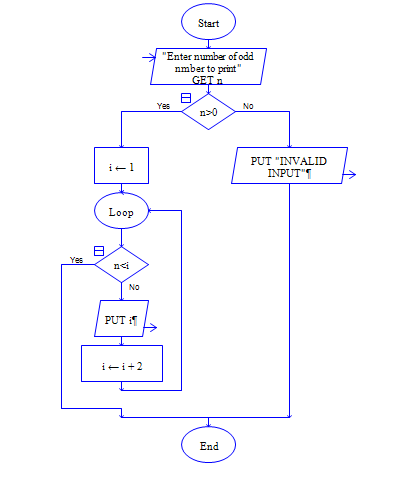# Java Program to print ODD numbers till N

Get input n and print n odd numbers.

Sample Input 1:

7

Sample Output 1:

1 3 5 7 9 11 13

#### Flow Chart Design#### Program or Solution

``` import java.util.*; class NOdd { public static void main(String args[]) { int i,lim; System.out.println("Enter The Value Of N"); Scanner sc=new Scanner(System.in); lim=sc.nextInt(); System.out.println("The N Odd Numbers: "); for(i=1;i<=lim;i=i+2) { System.out.println(""+i); } } } ```

#### Program Explanation

1. Get the input lim(Till which number the odd numbers to be printed)

2. Instruction(s) inside the for block{} are executed repeatedly till the second expression (i<=lim) is true.

for(i=1;i<=lim;i=i+2)

3. Here i is initialized to 1 and i is incremented by 2 for each iteration, instructions inside the for block are                  executed in every iteration. Iteration stops when i becomes greater than lim.

so value of i (1,3,5,7.....) (odd numbers till lim) in each iteration will be printed using system.out.println